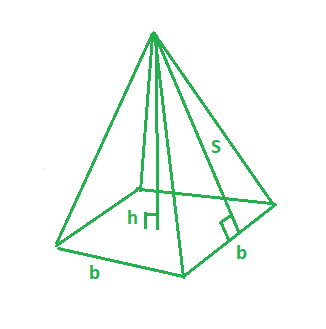# Program to find the surface area of the square pyramid

Given the base length(b) and slant height(s) of the square pyramid. The task is to find the surface area of the Square Pyramid. A Pyramid with a square base, 4 triangular faces, and an apex is a square pyramid.In this figure,
b – base length of the square pyramid.
s – slant height of the square pyramid.
h – height of the square pyramid.

Examples:

Input: b = 3, s = 4
Output: 33

Input: b = 4, s = 5
Output: 56


## Recommended: Please try your approach on {IDE} first, before moving on to the solution.

Formula for calculating the surface are of the square pyramid with (b) base length and (s) slant height.Below is the implementation using the above formula:

## C++

 // CPP program to find the surface area  // Of Square pyramid  #include  using namespace std;     // function to find the surface area  int surfaceArea(int b, int s)  {         return 2 * b * s + pow(b, 2);  }     // Driver program  int main()  {      int b = 3, s = 4;         // surface area of the square pyramid      cout << surfaceArea(b, s) << endl;      return 0;  }

## Java

 // Java program to find the surface area  // Of Square pyramid     import java.io.*;     class GFG {        // function to find the surface area  static int surfaceArea(int b, int s)  {         return 2 * b * s + (int)Math.pow(b, 2);  }     // Driver program            public static void main (String[] args) {                 int b = 3, s = 4;         // surface area of the square pyramid      System.out.println( surfaceArea(b, s));      }  }  //This code is contributed by anuj_67..

## Python 3

 # Python 3 program to find the   # surface area Of Square pyramid     # function to find the surface area  def surfaceArea(b, s):         return 2 * b * s + pow(b, 2)     # Driver Code  if __name__ == "__main__":      b = 3     s = 4        # surface area of the square pyramid      print(surfaceArea(b, s))     # This code is contributed   # by ChitraNayal

## C#

 // C# program to find the surface   // area Of Square pyramid  using System;     class GFG   {     // function to find the surface area  static int surfaceArea(int b, int s)  {         return 2 * b * s + (int)Math.Pow(b, 2);  }     // Driver Code  public static void Main ()   {      int b = 3, s = 4;             // surface area of the square pyramid      Console.WriteLine(surfaceArea(b, s));  }  }     // This code is contributed   // by inder_verma

## PHP

 

Output:

33


Don’t stop now and take your learning to the next level. Learn all the important concepts of Data Structures and Algorithms with the help of the most trusted course: DSA Self Paced. Become industry ready at a student-friendly price.

My Personal Notes arrow_drop_upCheck out this Author's contributed articles.

If you like GeeksforGeeks and would like to contribute, you can also write an article using contribute.geeksforgeeks.org or mail your article to contribute@geeksforgeeks.org. See your article appearing on the GeeksforGeeks main page and help other Geeks.

Please Improve this article if you find anything incorrect by clicking on the "Improve Article" button below.

Improved By : vt_m, inderDuMCA, chitranayal